## research papersJOURNAL OFSYNCHROTRONRADIATION
ISSN: 1600-5775
Volume 14| Part 6| November 2007| Pages 471-476

## Observation of interference effects in coherent diffraction of nanocrystals under X-ray standing-wave illumination

aLondon Centre for Nanotechnology, Department of Physics and Astronomy, Gower Street, London WC1E 6BT, UK, and bDepartment of Physics, University of Illinois, 1110 West Green Street, Urbana, IL 61801, USA
*Correspondence e-mail: i.robinson@ucl.ac.uk

(Received 5 April 2007; accepted 13 August 2007)

Coherent X-ray diffraction is a useful technique for understanding the structure of compact objects including those which can be represented as phase objects. X-rays are highly penetrating and have wavelengths very close to atomic spacing. In this work, gold nanocrystals (on a reflecting substrate) were imaged at the Advanced Photon Source and found to produce a novel double diffraction pattern. Simulations were carried out to explain the experimental diffraction pattern in terms of reflection of the incident beam from the substrate to produce a standing wave. The experimental data were then phased to produce a two-dimensional real-space image of the gold. It is expected that the standing-wave illumination may be a useful tool to aid the convergence of the phasing algorithms for nanocrystals.

### 1. Introduction

Many problems in science involve developing and exploring structures on the nanoscale, motivated by the interesting physical properties exhibited by materials in this size regime. Information regarding the size, density distribution, absolute free energy and structure of materials at this scale yield insights into the materials' behaviour, promising a new class of designer materials. However, obtaining a full three-dimensional structure of such an object can be extremely challenging.

X-rays are generally useful for looking at structure on the atomic scale, having a wavelength close to typical atomic spacings whilst also being very penetrating. While imaging with X-rays is very appealing, there is a lack of suitable lenses available to obtain the very high magnification needed. One solution has been to abandon the use of a lens altogether and employ computational methods instead, yielding a lensless X-ray method called coherent X-ray diffraction (CXD). One advantage is that diffraction from a crystal lattice acquires additional phase whenever atoms are displaced from a lattice site so that strain fields can be mapped, as demonstrated recently by Pfeifer et al. (2006).

Illuminating a small object with a coherent X-ray beam results in a far-field pattern which, when sampled in two dimensions (2D), is related to the Fourier transform of the projection of the density of the object onto a plane perpendicular to the exiting diffracted wavevector (Robinson & Miao, 2004; Livet, 2007). This simple relationship would allow for an inverse transform to image the object if the lost phase information could be recovered. This is the essence of coherent X-ray diffractive imaging (CXDI).

If we consider a collection of electrons to be a continuous charge density, ρ(r), illuminated by a wave ki, the scattered radiation is the superposition of the radiated fields from each volume element at the point of observation in direction kf. By making the kinematical approximation, whereby we assume that each photon only interacts once with the charge distribution and the incident wave is a plane wave, the scattered amplitude can be written as (Warren, 1990; Williams, 2004; Als-Nielsen & McMorrow, 2001)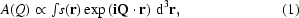where Q = kfki is the momentum transfer. The shape function s(r) can be a complex function, s(r) = |s(r)|exp(iφ), with the phase angle φ containing information about the strain within the crystal.

The CXD pattern is collected by means of a 2D charge-coupled device (CCD) detector, with the detector plane oriented perpendicular to the scattered wavevector kf. The x and y axes are chosen to lie in the plane of the detector and the z axis in the direction of kf. Therefore a 2D slice though a CXD pattern is related to the Fourier transform of the projection of the real-space density onto the xy plane. In this paper we only consider the measurement of such 2D patterns and the reconstruction of the corresponding 2D projections. Since it is the intensity I(Q) = |A(Q)|2 that the CCD measures, the phase of A(Q) is lost and must be recovered by computation before the Fourier transform can be inverted to obtain an image of the electron density ρ(r).

### 2. Experiment

The synthesis of gold nanocrystals involved the evaporation of gold onto a silicon dioxide substrate, forming a 20 nm polycrystalline layer. This was then heated to the melting point of gold (1337 K), causing it to de-wet from the surface of the substrate and form small molten droplets. These were then allowed to cool slowly to room temperature over a period of 12 h, giving plenty of time for gold nanocrystals to form. An empirical ratio of 1:10 exists for the deposition thickness to nanocrystal size. Hence nanocrystals in the range 100–200 nm were formed, with a random crystallographic orientation with respect to the substrate. The separation between the nanoparticles is approximately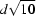, where d is the diameter of the nanocrystals. Hence for a deposition of 20 nm, the nearest-neighbour distance for 200 nm nanocrystals is approximately 600 nm.

The λ = 1.38 Å beam was prepared with a double-crystal Si(111) monochromator, focused to a probe of about 1 µm diameter by Kirkpatrick–Baez mirrors. The incidence angle of the beam onto the substrate was carefully controlled below the critical angle of the substrate (αC ≃ 0.2°). Multiple accumulations of the diffraction pattern were taken to reduce the saturation of the CCD. Unlike forward small-angle X-ray scattering experiments, CXD does not require a beamstop as the CCD is never exposed to the beam directly; only the diffracted and reflected-then-diffracted parts of the beam are measured.

Placing the gold-nanocrystal-coated substrate (described above) in the grazing-incidence X-ray beam, finding a crystal and moving the detector to a 111 Bragg point yielded the diffraction patterns shown in Fig. 1. A striking doubled peak intensity distribution was found under these conditions, the explanation of which is the main purpose of this paper. The beam diameter was 1 µm, leaving an oval optical footprint of approximately 100 µm × 1 µm on the sample. For 200 nm gold nanocrystals with a mean spacing of 600 nm, the beam will illuminate approximately 250 nanocrystals. All the nanocrystals have different crystallographic orientations with respect to the substrate. The CCD detector subtends about 1° of the diffraction cone and also about 1° of the rocking curve. Therefore the probability of seeing a diffracting nanocrystal is 250/(360)2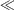1. This means that, despite the illumination of many gold nanocrystals, it is relatively rare to obtain the diffraction pattern of a single gold nanocrystal; two at the same time, as might explain the doublet in Fig. 1, would be highly unlikely. Indeed, imaging two nanocrystals with similar crystallographic orientations at the same time would introduce a strong speckle pattern caused by interference between their corresponding diffraction patterns, which is not seen.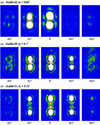Figure 1 CXD measurements of the paired diffraction peaks arising from the standing-wave illumination at grazing incidence. Each series represents numbered frames taken from a rocking scan, passing through the centre of the Bragg peak. The values of the rocking angle, θ, are indicated below each panel. The label refers to the data set as referenced in the text. The top two rows are on the same crystal with different incidence angles αi, while the bottom row is a different crystal.

Fig. 1shows measured 111 diffraction patterns, denoted Au606_51, Au606_49 and Au606_62, the first two taken from the same nanocrystal but with different angles of incidence, αi, of the incident beam onto the SiO2 substrate. As αi was increased up to the critical angle, the separation of the two primary peaks was found to increase in proportion, suggesting involvement of the totally reflected beam generated under these circumstances. As αi was increased beyond αC, the pattern generated by the reflected beam would vanish, leaving only the diffraction pattern from the direct beam. Many crystals were examined and were always found to show the same magnitude and direction separation of their doubled diffraction patterns, for a given αi.

Each diffraction image produced shows two central peaks surrounded by circular fringes, which exhibit threefold modulation. At each incidence angle the nanocrystal was rocked about the surface normal, which causes the 111 peak to pass through the Ewald sphere producing the series of frames illustrated. However, there is a central area of cancellation between the two central peaks in Au606_62 compared with a maximum in Au606_49 and multiple fringes in Au606_51. Since the intensity distribution is related to the square of the Fourier transform of the crystal shape, we can identify the prominent features of the patterns. The diffraction pattern resembles two copies of the spherical Bessel function, which is the Fourier transform of a sphere. File Au606_62 was chosen for further analysis, owing to its unusual central cancellation, the closeness of its Bragg peaks and its symmetry.

### 3. Standing-wave illumination

To support the experimental work, a theoretical model of the system was created to simulate the diffraction pattern. Simulations were written in C and called using Python scripts. A real-space array of density pixels was created and the diffraction pattern calculated using a fast Fourier transform (FFT). The nanocrystal is illuminated with a beam of coherence length greater than that of the object, allowing for the beam to be treated as coherent. The incident beam reflects from the substrate, at an angle below the critical angle of αC ≃ 0.2° as shown in Fig. 2(a). The nanocrystal object is considered to be illuminated by two incident waves, k1 and k2, which interfere with each other to create a standing wave. Referring to Fig. 2(b),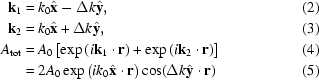represents the amplitude of the superposition of the two waves. Interference leads to a spatial modulation of the illuminating wavefield perpendicular to the reflecting surface with a period 2π/Δk. For incidence angles in the range 0 < αi < αC, the minimum accessible period is 2π/Δk = λ/αC ≃ 40 nm. This conveniently matches the 200 nm size scale of the nanocrystals under investigation here, in the sense that anywhere from zero to five periods of the standing wave can be superimposed on the crystal.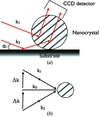Figure 2 (a) Schematic diagram of the experiment indicating how reflection from the substrate surface at grazing incidence results in a second incident beam reaching the sample. (b) Linear superposition of the two incident beams results in a standing-wave illumination field.

Diffraction patterns from a model nanocrystal were calculated assuming the standing wave form of the illumination function above. To work in 2D, the projected density of the crystal was modelled as the projection of a sphere, which is a circle of radius r0 with a density proportional to (r02-r2)1/2. Soft edges were also included to diminish the Bessel-like fringes in the diffraction pattern. The size of the model particle r0 and the FFT size were adjusted to match the pixel spacing of the experimental data of frame 23 of the selected data file Au606_62. After careful fitting, it was determined that the diameter of the nanocrystal was 170 ± 20 nm.

The resulting simulations are shown in Fig. 3. The amplitude of the illuminated object is striped by the presence of the standing wave, while the phase of the stripes is alternately 0 and π. The phase is represented as a cyclic function going from red, which represents a phase of zero, to green (π/2), to light blue (π), to dark blue (3π/2) and then back to red. Hence for the simulation in Fig. 3, which shows only red and light blue colours for phase, the object is entirely real, although the density does have positive and negative values. The value of Δk was found to determine the separation of the double diffraction peak, as expected. The chosen data showed a clear node of intensity along the bisector line of the two centres. The simulation could be improved by adding an additional phase shift, β, to the argument of the cosine term in (5). This phase shift β is an interesting variable in the experiment. It corresponds to the height of the nanocrystal above the reflecting surface. While the height cannot easily be varied experimentally, the wavelength of the standing wave, 2π/Δk, can be chosen using the incidence angle, αi. In this way the diffraction pattern can be tuned through the entire range of phase shifts, as long as there is sufficient separation between the crystal and the substrate mirror. Two extremes of this variation are illustrated in Fig. 3, varying from an antinode at β = 0 in Fig. 3(a)to a node at β = π/2 in Fig. 3(b). The latter case appears to agree better with experiment. This is also seen clearly in the data at different incidence angles shown in Fig. 1. The presence of a median node or antinode is readily seen in the data and can be used as an alignment aid.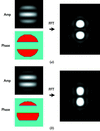Figure 3 (a) Simulation of the diffraction pattern obtained for the standing wave symmetrically illuminating a circular object (β = 0). (b) Simulation of the diffraction pattern obtained when the standing wave becomes antisymmetric with respect to the diffracting object (β = π/2).

It is also clear from the two examples of Fig. 3that the choice of β allows control of the symmetry of the sample being imaged. β = 0 results in illumination that is symmetric about the centre of the sample, while β = π/2 is antisymmetric. Values of 0 < β < π/2 would result in intermediate cases. This is potentially important in the phasing of diffraction patterns discussed in the next section. Fienup & Wackerman (1986) showed that the phasing algorithms are prone to stagnation' whereby a real-space image becomes confused with a twin' image, related by inversion symmetry. Either the object or its twin is a valid solution to the phasing problem, but a superposition of both is not, yet can often resemble one. The phasing algorithms can stagnate in a large number of possible superposition states and not find either of the extreme (untwinned) solutions. By using the standing-wave method described here, data can be deliberately chosen to correspond to the antisymmetric case, which is least likely to stagnate. Moreover, an entire range of illumination symmetries could be measured and employed to solve the phase problem self-consistently.

### 4. Phasing and inversion to images

According to (1), illuminating a small object with a coherent X-ray beam results in a far-field diffraction pattern, which is related to the Fourier transform of the projection of the density of the object onto a plane perpendicular to the exiting diffracted wavevector. This simple relationship would allow for an inverse transform to image the object in 2D. However, it is only the amplitude of the complex quantity A(Q) that is measured in the experiment, as its phase is lost. To recover the phase requires a solution of this phase problem'. According to the theoretical work mentioned below, if the pattern is oversampled in 2D there is sufficient redundancy in the formalism of the Fourier transform for a unique (beyond trivial symmetry) solution to exist. Finding that solution with clever algorithms is the essence of coherent X-ray diffractive imaging as explained by Williams (2004). Methods have been developed for microscopy through the application of iterative computational methods, which are the subject of this section.

The phasing algorithms used, function by successively applying real- and reciprocal-space constraints to an iterate. The earliest phasing algorithm was developed by Gerchberg & Saxton (1972) for extracting the missing phases in both real and reciprocal spaces when both amplitudes are known. Bates (1982) is attributed with showing that iterative phase retrieval works for reciprocal-space amplitudes only because generally there are very few sets of phases that can be matched to the measured amplitudes when a compact support is used for the real-space function. Mathematical proofs by Bruck & Sodin (1979), Sanz & Huang (1983) and the concept of factorisability by Barakat & Newsam (1984) support this idea. Two algorithms were used here, the error reduction (ER) based on the work of Gerchberg & Saxton (1972), and the hybrid input output (HIO), which was developed later by Fienup (1978).

The phasing methods depend on the data being oversampled relative to the spatial Nyquist frequency, because the phase information is effectively encoded in the amplitude of the diffraction fringes. Here we use data showing about 20 pixels per fringe, where the Nyquist frequency corresponds to two pixels per fringe. The oversampling ratio is therefore about ten, according to the definition of Miao et al. (1998). Oversampling-based phasing methods have been applied to a variety of systems, including arrays of gold balls by Marchesini et al. (2003), cut-out graphics using a soft X-ray free-electron laser by Chapman et al. (2006) and even freeze-dried whole biological cells by Shapiro et al. (2005).

Our implementation of the phasing algorithm involves the following steps. (i) Fourier transform an estimate of the real-space density. (ii) Make the smallest change possible to the Fourier transform, retaining its phase and overwriting its amplitude with the measured value. This is called the Fourier modulus constraint. (iii) Back transform the resulting estimate of the diffracted amplitude. (iv) Make the smallest changes possible to the calculated real-space density so that it obeys the real-space constraint to arrive at a revised estimate of the real-space density.

Most of the intelligence surrounding different algorithms involves the implementation of step (iv). In our version of ER, we set the density to zero outside a support' region, chosen to estimate the expected shape of the sought object. Inside the support, amplitude and phase are unchanged. In HIO, this same procedure is applied, with the exception that points outside the support are extrapolated by forming a linear combination with the previous iterate. In our experience the choice of the support is the most critical part of this procedure. It should be slightly larger than the object, but not cut it in any way. If it is too large, the convergence of the algorithm suffers and uniqueness becomes a problem: multiple solutions result from different choices of random numbers used to seed the algorithm. If the support is too large by a factor of two, the true object's autocorrelation function, which is twice as large, will form a solution, with all the diffraction phases the same.

We have verified that the doubled diffraction patterns can be phased in 2D by the application of support-based iterative algorithms. The result, shown in Fig. 4, compares images obtained by inverting both the measured data and the best-fit simulation described above. Inversion of the simulation yields a striped circular image, similar to that used to simulate the data in the first place. The asymmetry built into the phase is accurately reproduced in well defined colours in the phase map. The real data, which are expected to contain finer features not considered in the simulation, produced a similar image with some noteworthy differences: because the simulation is close to the ideally antisymmetric case (β = π/2), the two edge stripes in the amplitude of the simulated inversion are almost equally strong; in the inverted-data image, one side is suppressed. This is attributed to the fact that spherical crystals growing on a substrate are indeed expected to have a flat face of contact. Small undulations in the density distribution along the stripes, by comparison with the simulation, are considered to be due to projections of a slightly out-of-round shape and small internal lattice distortions.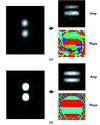Figure 4 Results from phasing and inversion of the diffraction patterns using 100 cycles of the ER algorithm, followed by 500 cycles of the HIO algorithm and 100 more cycles of the ER algorithm. (a) Using the measured diffraction pattern. (b) Using the simulated object that best explained those data.

### 5. Conclusions and outlook

We have demonstrated that doubled X-ray diffraction patterns of nanocrystals attached to a mirror surface can be attributed to the formation of a standing-wave illumination field. Simulations show that the standing wave can be manipulated in a useful way by varying the grazing incidence angle onto the mirror. The alternating sign of the illuminating field shows up faithfully in the images of the object after phasing and Fourier inversion by standard iterative methods. The ability to manipulate the illumination at the time of measurement by varying the angle of incidence could lead to significant improvements in the imaging methods.

Firstly, an antisymmetric object can be artificially created, which should greatly reduce the likelihood of stagnation of the phasing algorithms. Secondly, the known sinusoidal density distribution and phase alternation could be used as a powerful real-space support constraint to assist the phasing. Fienup (2006) has recently demonstrated coherent imaging by phase retrieval with an illumination pattern constraint. Lastly, a series of diffraction patterns can be collected as the standing wave is swept across the nanocrystal, allowing piecewise assembly of the structural density and possibly a self-consistent overall solution of the phase problem. Rodenburg et al. (2007) have demonstrated a working `ptychography' algorithm which cycles through diffraction patterns from a known series of overlapping illumination apertures on an extended object and solves for the phases using the redundancy of information; a series of standing-wave positions or spacings could be used with similar effect on a much finer length scale.

The images of a gold nanocrystal that we have obtained are dominated by the standing-wave effects, which we have explained, but also contain information about the structure itself. Small modulations in the density can be seen in Fig. 4, which may be density variations associated with defects. Pixels with phases other than 0 and π/2 can be seen near the nodes of the standing wave, but these may be affected by noise since the phase is undefined when the amplitude is zero. The effects of surface roughness of the reflector have not been investigated, but it had been observed that the reflected diffraction pattern fades out smoothly as the critical angle is crossed (for the incident beam). A very rough surface with little or no specular component would not be expected to show a double pattern, while its diffuse scattering would superimpose multiple images that would just tend to reduce the contrast. Finally, a curved or macroscopically distorted substrate would probably work fine, since a mirror is needed only in the immediate vicinity of the nanocrystal sample.

### Acknowledgements

This research was supported by EPSRC grant EP/D052939/1 and NSF DMR 0308660. The experimental work was carried out at the Advanced Photon Source (APS) beamline 34-ID-C, which was operated by the Materials Research Laboratory of the University of Illinois under DOE contract DEFG02-91ER45439. APS is operated by DOE contract No. W 31 109 ENG 38. PG acknowledges receipt of a summer bursary from the Nuffield foundation.

### ReferencesJOURNAL OFSYNCHROTRONRADIATION